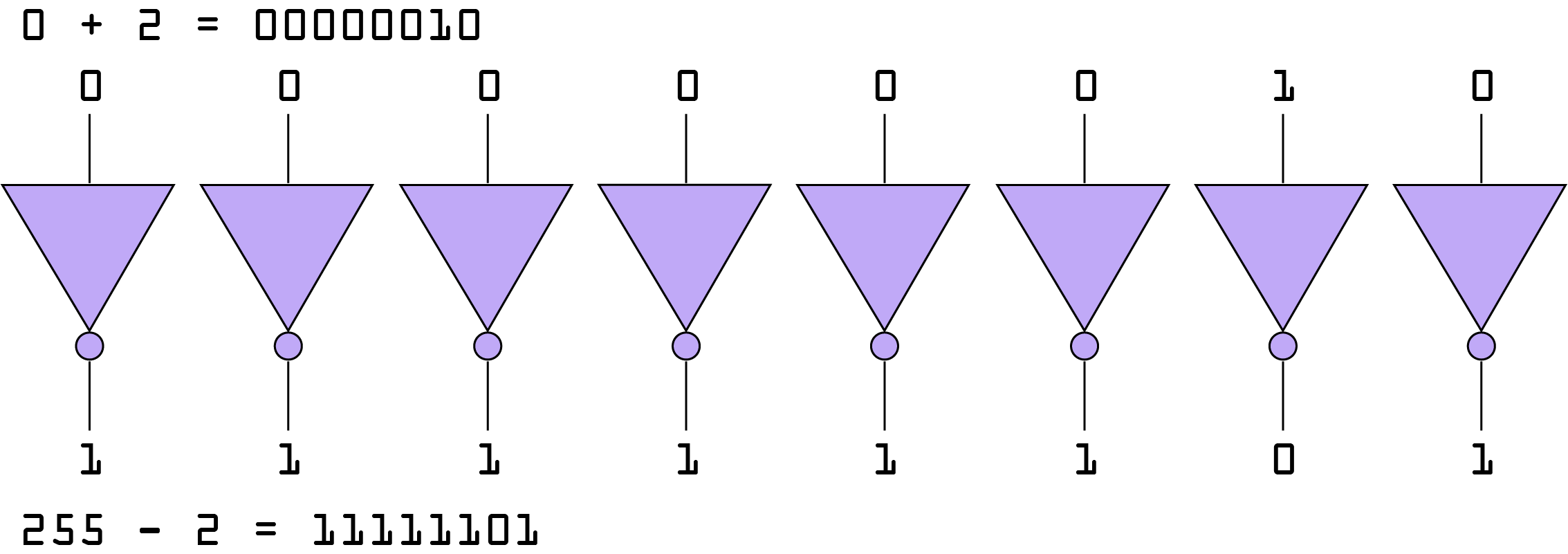# How I accidentally learned something unexpected from a simple binary counter

In my previous post, I talked about how I implemented a binary counter with my Mojo FPGA board.

I am working on a follow-up post on implementing a reverse binary counter with a switch that lets you change between the incrementing ( `UP` ) counter and the decrementing ( `DOWN` ) counter.

I accidentally discovered a property of 8 bit numbers. It's referred to as the "Ones' complement".

When you add a number n between 0 and 255 to an 8-bit binary representation of 0, the same number n subtracted from 255 will be the binary inverse (bit complement) of the first number.

To demonstrate, let's say we start with an 8-bit number at 0:

`00000000`

`00000010`

OK, let's look what happens when we have 255:

`11111111`

Now, let's subtract 2:

`11111101`

So, comparing them, one above the other, we can see that they are inverse:

``````00000010
11111101``````

This is true for all numbers between 0 and 255 (the only assertion I can personally make through observation).

So, in my original implementation of the reverse binary counter, I was using two counters to keep up with the numbers; one that started at 0 and counted up, and one that started at 255 and counted down.

As I switched between the two to test my design, I noticed the inversion almost immediately. This might be a neat trick when you want to flash LED's, too.

I think a more efficient implementation to replace the `DOWN` counter is to apply a simple inverter to each bit.ones' complement inverter for 8-bit number

This is what happens when you learn in reverse. Rather than simply being taught this in a ten minute aside from a lecture, I had to accidentally discover it. I'm learning electronics engineering from a hobbyists perspective, so I'm avoiding the theory and the complex math that makes it actual engineering. I'm sure I'll learn a lot more in the coming years.

This hobby never stops giving...